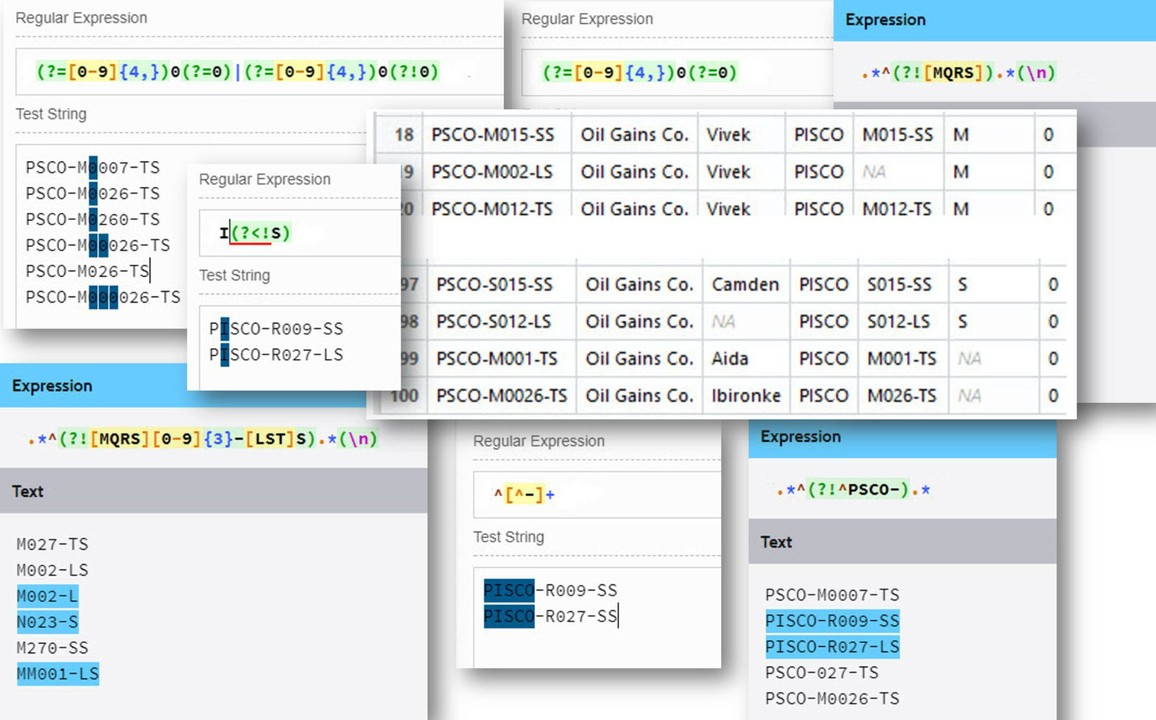Data Science for Petroleum Engineering - Part 5.3 Finding and filling missing well data in alphanumerics

Alfonso R. Reyes
(24 August 2017)

OnlineThis is what we will reviewing in this lecture.

NOTE. You can find the PDF version of the R markdown notebook in GitHub at this link. The reproducible R markdown notebook itself is here. Both are full versions of this LinkedIn article. For the time being, LinkedIn publishing does not support markdown which would make sharing scientific and engineering documents much easier.

``# code``

We will see that some well names can be fixed manually and others should be done automatically with a script.

In our particular case we only have 100 wells but what about if we have 1000, or 5000? Doing it manually is not an option. Some are quickly fixable some others are more challenging. Let’s start by the easier ones.

When correcting data, always go from the more general to the more particular. convert lowercase to uppercase Let’s convert the well names to uppercase and verify how many were corrected.

``# code``

Two were corrected.

removing spaces

``# code``

One well name was corrected.

correct the completion type The completion type, at the end of the well name, should have two characters: LS, TS or SS.

``# code``

Those were the easy ones. We had three corrections. There are 5 more to go.

correcting the field abbreviation in the well name There are two wells that were not properly field identified. We have an additional “I” in the field name abbreviation. We have to remove it. At this point we have two choices: (1) change all the first 4 first characters to PSCO, or, (2) replace only those two well names with the issue by replacing the “I” with a blank.

``# code``

In the example we used invert=TRUE to negate the correct pattern. If we want the regex pattern including the negation we would have to use:

``# figure``

option (1): change all the first 4 first characters to PSCO

``# code``
``# figure``
``# code``

option (2): replace only those two well names with the issue.

``# code``
``# figure``
``# code``

correct the length of the well number The well names have been corrected on the field identifier. Next if correcting the length of the well number.

``# code``

Alright. So far, we have corrected the field name in the well name. There are still three more wells to go which problems are:

``# text``

The well number should go from 000 to 999, right after the field identifier (one character).

``# code``

Replacing:

``# code``

Very good. Now we have one well left.

``````# code
# figure``````

If we had longer numbers we would modify the regex to:

``# figure``

See in this example that as more zeros show up in the number (last line), those zeros are removed from the string to fit the 3 digit number limit.

Add the one-letter platform identifier to the well name

``````# code
# figure``````
``# code``
``# code``

Check if Company is correct

``# code``

We don’t get any return. All the company names are the same. Cool!

Detect incorrect names and synonyms in Analyst

``# code``

We can correct manually. In this example we will make use of the operator %in%. It is pretty handy for checking if elements belong to a particular group.

``# code``

There is only one observation left, the one with NA. We will have to cross-reference it.

Find and replace incorrect and missing values in Field

``# code``

It has been fixed now.

Add a column for the Completion type To close this chapter, let’s add a new variable (column) where we have only the Completion Type. We can take advantage that the last two characters of the well name is the completion type.

We introduce here another function nchar which returns the number of characters of a string of text. The second function is substr.

``# code``

Let’s apply these two functions:

``# code``

Replace values in Location

``# code``

Observe that in this example we are using the pattern [MQRS][0-9]{3}-[LTS]S together with the parameter invert=TRUE in grep. This means that the pattern will be negated when invert is TRUE.

``# code``

If we would like instead is the regex for the negated pattern it would have to look like this:

``# figure``

You see that the words matched are those which do not match the correct pattern.

Replace NA values in Platform

``# code``

Again, if instead of using invert=TRUE in grep we could have used the negation of the pattern which is:

``# figure``

What this regex does is match those words that do not contain a valid platform character.

``# code``